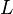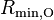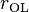# SWM4-DP model of water

The SWM4-DP model   of water has a TIP4P like structure, as well as incorporating a Drude oscillator.

## Parameters$r_{\mathrm {OH}}$ (Å)$\angle$HOH (deg)$\sigma$ (Å)$\epsilon$ (kcal/mol) q(O) (e) q(H) (e) q(M) (e)$r_{\mathrm {OM}}$ (Å) q(Drude) (e) k(Drude) (kcal/mol/Å2) 0.9572 104.52 3.1803 0.20568 -1.77185 0.5537 -2q(H) 0.23808 -q(O) 1000

## SWM4-NDP

The SWM4-NDP model is a version that has been re-optimized for negatively charged Drude particles. The parameters are given by:$r_{\mathrm {OH}}$ (Å)$\angle$HOH (deg)$\sigma$ (Å)$\epsilon$ (kcal/mol) q(O) (e) q(H) (e) q(M) (e)$r_{\mathrm {OM}}$ (Å) q(Drude) (e) k(Drude) (kcal/mol/Å2) 0.9572 104.52 3.18395 0.21094 1.71636 0.55733 -2q(H) 0.24034 -q(O) 1000

## SWM6

The SWM6  model is a six-site model that adds oxygen lone-pairs ($L$) to the SWM4-NDP model. The parameters are given by:$r_{\mathrm {OH}}$ (Å)$\angle$HOH (deg)$\angle$LOL (deg)$R_{ \mathrm{min, O} }$ (Å)$\epsilon$ (kcal/mol) q(O) (e) q(H) (e) q(M) (e) q(L) (e)$r_{\mathrm {OM}}$ (Å)$r_{\mathrm {OL}}$ (Å) q(Drude) (e) k(Drude) (kcal/mol/Å2) 0.9572 104.52 101.098 3.59 0.162 1.91589 0.53070 -1.1334 -0.108 0.247 0.315 -1.62789 1000